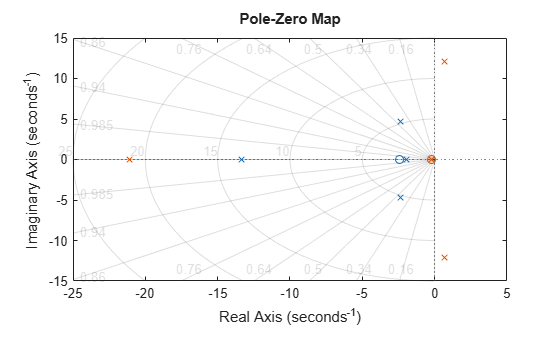Main Content

# Pole and Zero Locations

This example shows how to examine the pole and zero locations of dynamic systems both graphically using `pzplot` and numerically using `pole` and `zero`.

Examining the pole and zero locations can be useful for tasks such as stability analysis or identifying near-canceling pole-zero pairs for model simplification. This example compares two closed-loop systems that have the same plant and different controllers.

Create dynamic system models representing the two closed-loop systems.

```G = zpk([],[-5 -5 -10],100); C1 = pid(2.9,7.1); CL1 = feedback(G*C1,1); C2 = pid(29,7.1); CL2 = feedback(G*C2,1);```

The controller `C2` has a much higher proportional gain. Otherwise, the two closed-loop systems `CL1` and `CL2` are the same.

Graphically examine the pole and zero locations of `CL1` and `CL2`.

```pzplot(CL1,CL2) grid````pzplot` plots pole and zero locations on the complex plane as `x` and `o` marks, respectively. When you provide multiple models, `pzplot` plots the poles and zeros of each model in a different color. Here, there poles and zeros of `CL1` are blue, and those of `CL2` are green.

The plot shows that all poles of `CL1` are in the left half-plane, and therefore `CL1` is stable. From the radial grid markings on the plot, you can read that the damping of the oscillating (complex) poles is approximately 0.45. The plot also shows that `CL2` contains poles in the right half-plane and is therefore unstable.

Compute numerical values of the pole and zero locations of `CL2`.

```z = zero(CL2); p = pole(CL2);```

`zero` and `pole` return column vectors containing the zero and pole locations of the system.

## Support

#### Learn how to automatically tune PID controller gains

Download code examples